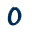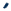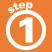Home    |    Teacher    |    Parents    |    Glossary    |    About UsIn our decimal number system, the value of a digit depends on its place, or position, in the number. Each place has a value of 10 times the place to its right.

A number in standard form is separated into groups of three digits using commas. Each of these groups is called a period.

Click the Start, Stop, and Reset buttons on the digital counter below to understand more about how the place value works.Homework Help | Pre-Algebra | NumbersEmail this page to a friendSearch·  Place value·  Decimal numbers·  Estimating and     rounding·  Adding / subtracting     decimals·  Multiplying decimals·  Dividing decimals·  Percent·  Exponents·  Square roots·  Signed integers·  Adding and     subtracting integers·  Multiplying and     dividing integers·  Properties of integersFirst Glance In Depth Examples WorkoutPlace value